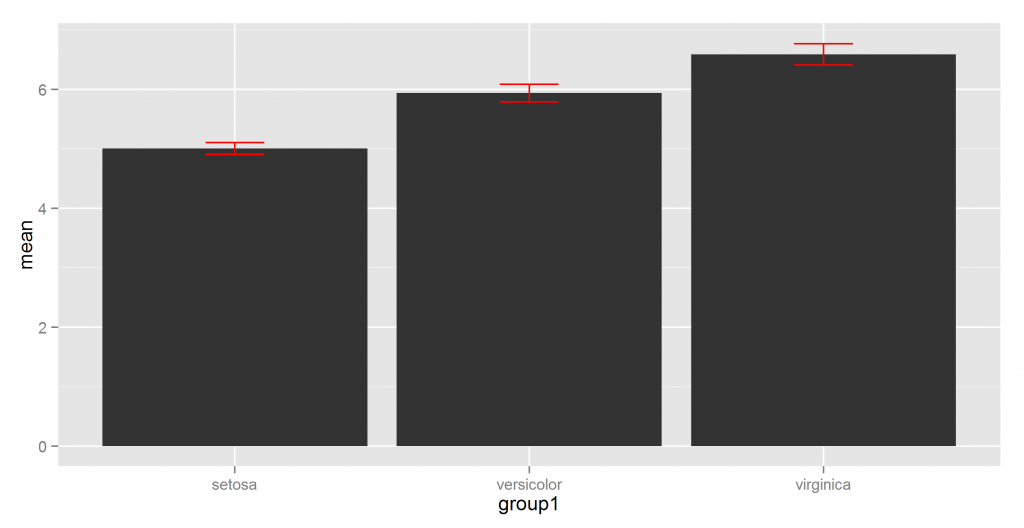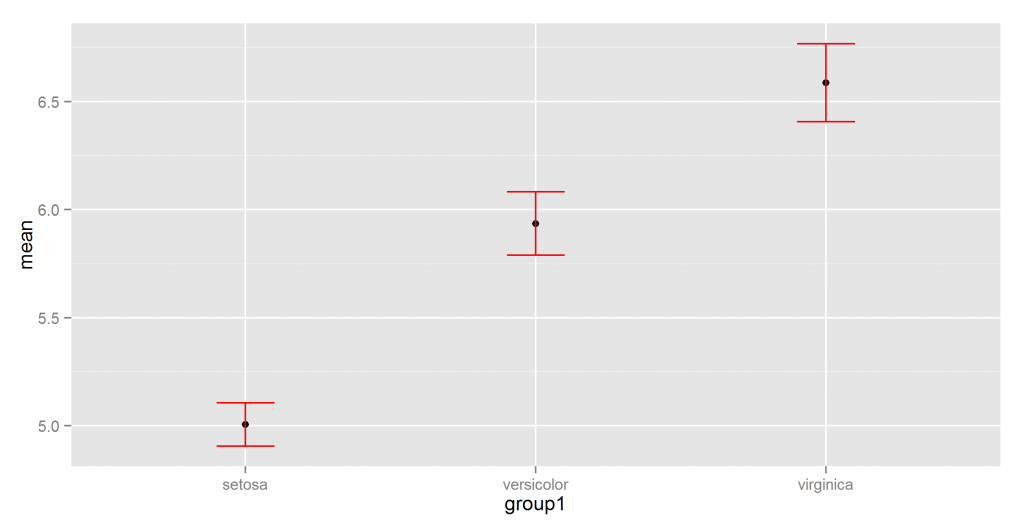## kirkegaard: GG_group_means(), easy plotting of group means using ggplot2

Do you find yourself in need of making nice ggplot2 plots for group means over and over again? Are you tired of (re-)writing big chunks of code for something that should be easy? Here’s a solution. First install the package from Github using devtools:

```library(devtools)
install_github("deleetdk/kirkegaard")```

Then we plot:

```library(kirkegaard)
GG_group_means(iris, "Sepal.Length", "Species")```But what if we don’t like bar plots? Maybe because values of 0 don’t make sense:

`GG_group_means(iris, "Sepal.Length", "Species", type = "point")`But what if we think that using just one point obscures the internal variation in groups? Let’s use all the points:

`GG_group_means(iris, "Sepal.Length", "Species", type = "points")`But what if we really, really want to be sure of getting the right means? Maybe use a 99.9999% confidence interval:

`GG_group_means(iris, "Sepal.Length", "Species", type = "points", CI = .999999)`